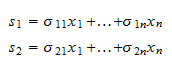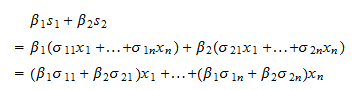StatLect

# Linear span

The span of a set of vectors, also called linear span, is the linear space formed by all the vectors that can be written as linear combinations of the vectors belonging to the given set.## Definition

Definition Letbevectors. Their linear span is the setof all the linear combinationsthat can be obtained by arbitrarily choosingscalars, ...,.

A very simple example of a linear span follows.

Example Letandbecolumn vectors defined as follows.Letbe a linear combination ofandwith coefficientsand. Then,Thus, the linear spanis the set of all vectorsthat can be written aswhereandare two arbitrary real numbers. In other words,.

## A linear span is a linear space

The following proposition, although elementary, is extremely important.

Proposition The linear span of a set of vectors is a linear space.

Proof

Letbe the linear span ofvectors. Then,is the set of all vectorsthat can be represented as a linear combinationTake two vectorsandbelonging to. Then, there exist coefficientsandsuch thatThe spanis a linear space if and only if for any two coefficientsandthe linear combinationalso belongs to. But,Thus, the linear combinationcan itself be expressed as a linear combination of the vectorswith coefficients, ...,. As a consequence, it belongs to the span. In summary, we have proved that any linear combination of vectors belonging to the spanalso belongs to the span. This means thatis a linear space.

The book

Most of the learning materials found on this website are now available in a traditional textbook format.

Glossary entries
Share